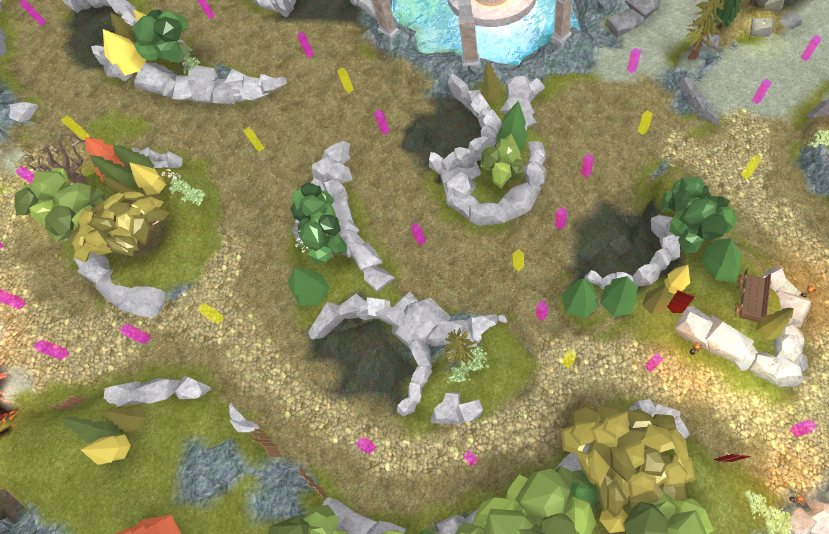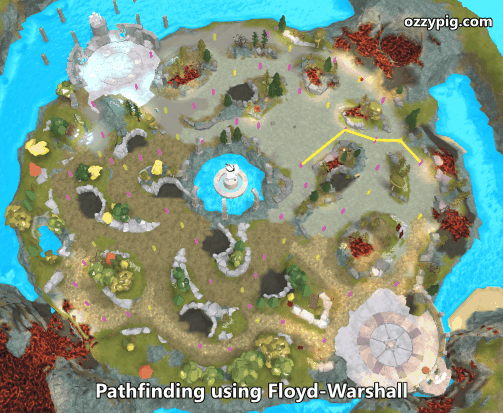# Graph Theory in Roblox (Part 3 – A Solution)

In the previous article, I described a problem I faced when developing a game with little dudes roaming around a pre-built map. In this article, I’ll outline how I applied graph theory (described in the first article) to solve my problem.

Graphs are about relationships between objects. On my map, there are places that I know a minion can be, and I know which of those places can be accessed from nearby places. I placed parts in Roblox Studio on my map to quickly identify such locations:Locations on the map that are navigable. Pink nodes represent a path; yellow represents a junction.

Inside each of these Parts, I added ObjectValues whose Values point to adjacent locations in which a minion should be able to travel between. This is essentially building an adjacency list, where each node contains a list of adjacent nodes. This is one of the two main ways to represent a graph in memory; the other method is to store an adjacency matrix of size n-by-n, where n is the number of nodes in a graph. However, I am working with a sparse graph, which means the 100+ nodes only connect to a select few (2-6) other nodes near to them.

Now I have a representation of my map’s network of navigable areas. At runtime, I have the game convert my Parts and ObjectValues into nodes and edges in a graph. The weights of the edges are the euclidean distance between the nodes. If you’d like to view my implementation of graph, node and edge objects, you can download an rbxmx file here. If you’d like to view the source code of each script individually, here are some pastebin links:

The algorithm I selected for shortest path calculation  is the Floyd-Warshall algorithm. For my fellow CS nerds, it runs in order n-cubed time (for every n nodes, we perform n×n×n steps). This algorithm finds all possible paths between all nodes and leaves each node a list of nodes one should travel to if they are to reach a specific destination node. In other words, imagine an n-by-n table, on the left is your current node, on the top is your destination node. It fills out the table with an adjacent node one ought to which one should travel.Using the results of the Floyd-Warshall algorithm to find the shortest path between two nodes on a graph.

Above is an animation of randomly chosen paths being rendered in sequence. Here is my implementation (also included in the rbxmx file above) as a mix-in for the Graph class:

The result is pretty awesome! Now, all I do is spawn minions at a random node and randomly choose another random node to path towards. And finally here’s a preview of the two minions using the generated paths.